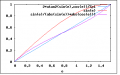# File:AngleApproximationQuality.png

(diff) ← Older revision | Latest revision (diff) | Newer revision → (diff)
Graph showing that $\displaystyle{ \frac{y}{|x|+|y|} }$ gives a (much) better estimate of the angle than a sine ($\displaystyle{ \frac{y}{\sqrt{x^2+y^2}} }$). Note that the blue line is the ideal line.
current12:05, 17 April 2009412 × 257 (4 KB)Jaspervdg (talk | contribs)Graph showing that $\frac{y}{|x|+|y|}$ gives a (much) better estimate of the angle than a sine ($\frac{y}{\sqrt{x^2+y^2}}$).